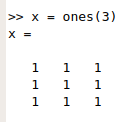# IntroductionFirst of all, let me tell you this is part of the Coursera Machine Learning course. Here I will show how to implement a Neural Network Backpropation in Octave. I know there are already several options to do this in a very high level (TensorFlow), just focusing on inputs and outputs, but I would like to understand all the matrix and statistical fundamentals to later be able to choose the right strategies in high level programs. Backpropagation algorithm is a guided learning algorithm, i.e., we need to traning the Neural Network with real outputs in order to obtain good predictions.

# Initialize variables

## Initialize matrix with ones# IntroductionWhen we playing we see moving objects around the scenery, so today let's have a look how one of the most important pieces of that movement works: translation. There are many ways to perform the same maths, but the GPU must follow the defined pipeline and that's way we use matrices.Sometimes matrix multiply operations are hard to see and understand. With this small application done in C++ in Qt I try to show how a matrix multiplication of range equal three is done.